# KSEEB Class 10 Science Important Questions Chapter 13 Magnetic Effects of Electric Current

Students can download Class 10 Science Chapter 13 Magnetic Effects of Electric Current Important Questions, KSEEB SSLC Class 10 Science Important Questions and Answers helps you to revise the complete Karnataka State Board Syllabus and score more marks in your examinations.

## Karnataka SSLC Class 10 Science Important Questions Chapter 13 Magnetic Effects of Electric Current

Question 1.
What is magnetic effect of electric current? Who discovered this phenomenon?
A current flowing through a conductor produces a magnetic field in the region surrounding the conductor. This phenomenon is known as magnetic effect of electric current. H.C. Oersted discovered the phenomenon of magnetic effect of electric current.

Question 2.
Describe an experiment to show the magnetic effect of electric current.
Take a straight thick copper wire AB. Connect the ends of the conductor in series with a battery and a tap key as shown in the figure. Place a small compass needle horizontally just below the copper wire. Note the position of its needle. Now, pass current through the circuit by closing the key. Suddenly we see a deflection in the compass needle.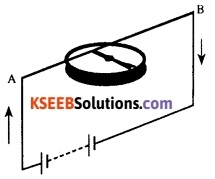On stopping the current, the needle comes back to its original direction. On reversing the direction of current, the direction of deflection of the compass needle will also get reversed. This means that the electric current flowing through the copper wire has produced a magnetic effect.Question 3.
How many magnetic poles does a magnet usually have? Name them.
A magnet usually has two magnetic poles: a north pole and a south pole.

Question 4.
How do you identify the north pole and the south pole of a bar magnet or a compass needle?
When a bar magnet is suspended freely, it aligns itself approximately in north-south direction. Similarly, the needle of a compass aligns itself in north-south direction. The end of the magnet that points in the southern direction is called the south pole and the end that points in the northern direction is called the north pole of the magnet.

Question 5.
State the rule regarding attraction and repulsion between magnetic poles.
The rule regarding magnetic poles states that ‘like magnetic poles repel each other and unlike magnetic poles attract each other.’

Question 6.
Why does a compass needle set deflected when brought near a bar magnet?
A compass needle is a small bar magnet. When it is brought near a bar magnet, its poles interact with those of the bar magnet. Like magnetic poles repel and unlike magnetic poles attract. Hence, a compass needle shows a deflection when brought near the bar magnet.

Question 7.
What is magnetic field?
The region surrounding a magnet where the influence of the magnet can be experienced is called a magnetic field. It is the region where a magnetic force exists.Question 8.
Why is magnetic field called a vector quantity?
Magnetic field has both magnitude and direction and we need to mention both for its complete specification. This is why magnetic field is called a vector quantity.

Question 9.
What is a magnetic line of force?
The path taken by an imaginary unit north pole in a magnetic field is called a magnetic line of force. .

Question 10.
What is the direction of a magnetic line of force?
A magnetic line of force always moves away from North Pole and moves towards South Pole.

Question 11.
Draw magnetic field lines around a bar magnet.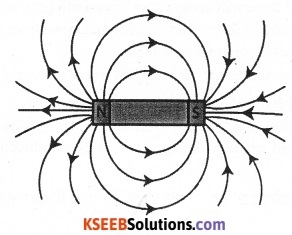Question 12.
How do you obtain the pattern of magnetic lines of force around a bar magnet using iron filings?
Fix a sheet of white paper on a drawing board using some adhesive. Place a bar magnet in the centre. Sprinkle some iron filings uniformly around the bar magnet. Now tap the board gently. The iron filings arrange themselves in a pattern shown in the figure along the magnetic field lines. This will give us an idea of the pattern of the magnetic field around a bar magnet.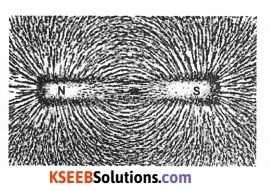Question 13.
List two methods of producing magnetic field.
Three methods of producing magnetic field are as follows:

1. Magnetic field can be produced by placing a permanent magnet or a horse-shoe magnet at the place where magnetic field is required.
2. Magnetic field is produced around a current carrying straight conductor or a current carrying coil.
3. Magnetic field is also produced due to flow of current in a solenoid.Question 14.
How can we obtain the pattern of magnetic lines of force around a bar magnet using a compass needle?
Take a small compass and a bar magnet. Fix a drawing sheet on a table with the help of drawing pins. Place the bar magnet in the middle and mark the boundary of the magnet. Place the compass near the north pole of the magnet. The south pole of the needle points towards the north pole of the magnet and the north pole of the compass is directed away from the north pole of the magnet. Mark the position of the two ends of the needle.

Now move the needle to a new position such that its south pole occupies the position previously occupied by its north pole. In this way, proceed step by step till you reach the south pole of the magnet. Join the points marked on the paper by a smooth curve.

This curve represents a field line. Repeat the procedure above and draw as many lines as possible on either side of the magnet. The lines represent the magnetic field around the magnet. These are known as magnetic field lines.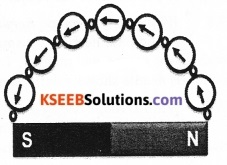Question 15.
List the properties of magnetic lines of force.

OR

Mention the properties of magnetic field lines.
The following are the properties of magnetic lines of force:

1. The magnetic lines of force will always start from north pole and end at south pole.
2. The direction of the lines of force inside the magnet move from south pole towards north pole.
3. Magnetic lines of force never cross each other.
4. Magnetic lines of force are denser in the region where magnetic field is stronger.

Question 16.
Why don’t two magnetic lines of force intersect each other?
No two magnetic field-lines are found to cross each other. If they did, it would mean that at the point of intersection, the compass needle would point towards two directions at the same time, which is impossible.

Question 17.
The magnetic field in a given region is uniform. Draw a diagram to represent it.
Uniform magnetic field: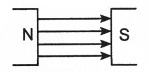The field lines in a uniform magnetic field arc parallel straight lines. In such a field, an isolated unit north pole will experience the same force in the same direction at all points.Question 18.
Draw a neat diagram showing the pattern of concentric circles indicating the field lines of a magnetic field around a straight conducting wire.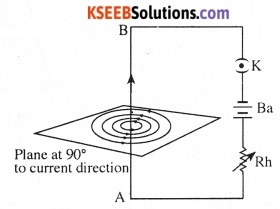Question 19.
Describe an experiment to obtain the pattern of the magnetic field around a straight conductor carrying current. Describe the pattern.
Take a rectangular cardboard having a small hole in the middle. Insert a straight conductor AB through the hole. Connect the conductor in series with a battery, a key and a rheostat. Sprinkle iron filings uniformly on the cardboard.

Ensure that the conductor is at right angles to the plane of the cardboard. Now close the key and pass current through the conductor. Gently tap the cardboard a few times. Iron filings arrange themselves along the field lines. The circles are found to become larger as we move away from the conductor.

The magnetic lines of force around a straight conductor carrying current are in the form of concentric circles with the conductor as centre. The direction of the magnetic lines of force can be known by the Right Hand Thumb Rule.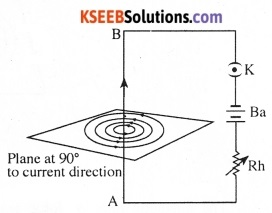Question 20.
State Right Hand Thumb Rule.

OR

Draw the diagram showing the magnetic field lines around a current-carrying conductor. Label the following:

• The direction of magnetic field lines.
• The direction of electric current.

Right Hand Thumb Rule: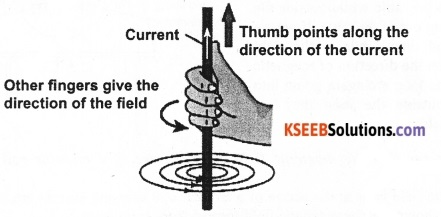Imagine you are holding a current-carrying straight conductor in your right hand such that the thumb points towards the direction of current. Then, the fingers will wrap around the conductor in the direction of the field lines of the magnetic field. This is known as Right Hand Thumb Rule.Question 21.
A current through a horizontal power line flows in east to west direction. What is the direction of magnetic field at a point directly below it and at a point directly above it?
The current is in the east-west direction. Applying the right-hand thumb rule, we get that the magnetic field (at any point below or above the wire) turns clockwise in a plane perpendicular to the wire, when viewed from the east end, and anti-clockwise, when viewed from the west end.

Question 22.
Describe an experiment to show the pattern of the magnetic field around a circular conductor carrying current.
Take a rectangular cardboard having two small holes in it. Insert a circular coil of wire through the two holes. Connect the ends of the coil in series with a battery, a key and a rheostat. Sprinkle iron filings uniformly on the cardboard.

Ensure that the plane of the cardboard is at right angles to the plane of the coil. Pass current through the coil by closing the key. Gently tap the cardboard a few times. The iron filings arrange themselves along the field lines in a pattern shown in the figure.The magnetic lines of force around a circular conductor carrying current are in the form of concentric circles near the conductor and are in the form of parallel straight lines at the centre of the circular coil. The direction of the lines of force can be known by the Right Hand Thumb Rule.

Question 23.
Consider a circular loop of wire Ivins in the plane of the table. Let the current pass through the loop clockwise. Apply the right-hand rule to find out the direction of the magnetic field inside and outside the loop.
In the diagram the current is flowing clockwise. If we are applying right hand thumb rule to the left side of the loop then the direction of magnetic field lines inside the loop are going into the table while outside the loop they are coming out of the table. If we are applying right hand thumb rule to the right side of the loop then the direction of magnetic field lines inside the loop are again going into the table while outside the loop they are coming out of the table.Question 24.
Why do we say that the magnetic field at the centre of a circular coil carrying current is uniform?
The magnetic field lines at the centre of a circular coil carrying current are in the form of parallel straight lines. This shows that the magnetic field formed there is uniform.

Question 25.
What are the factors on which the field around a circular conductor carrying current depends?
The magnetic field around a circular conductor carrying current depends on:

1. The amount of current flowing through the wire.
2. Radius of the circular wire.
3. Number of turns in the circular wire.

Question 26.
How can the strength of the magnetic field produced by a current-carrying circular coil be increased?
The strength of the magnetic field produced by a current-carrying circular coil can be increased by

1. Increasing the number of turns of wire in the coil.
2. Increasing the current flowing through the coil.
3. Decreasing the radius of the coil.

Question 27.
What is a solenoid? Draw a neat diagram showing the pattern of the magnetic field around a current carrying solenoid. Describe the pattern of the magnetic field produced around a current carrying solenoid.
A coil of many circular turns of insulated copper wire wrapped closely in the shape of a cylinder is called a solenoid. The pattern of the magnetic field lines around a current-carrying solenoid is similar to the magnetic field around a bar magnet.

In fact, one end of the solenoid behaves as the magnetic north pole, while the other behaves as the south pole. The field lines outside the solenoid move away from the end that behaves like north pole towards the end that behaves like south pole.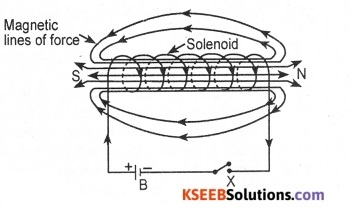The field lines inside the solenoid are in the form of parallel straight lines. This indicates that the magnetic field is the same at all points inside the solenoid. That is, the field is uniform inside the solenoid. The field is stronger at the ends of the solenoid.Question 28.
How does a solenoid behave like a magnet? Can you determine the north and south poles of a current-carrying solenoid with the help of a bar masnet? Explain.
A solenoid begins to behave like a magnet when electric current flows through it. We know that any current-carrying conductor creates a magnetic field around it and that is what happens in the case of solenoid.

In order to determine the polarities of its ends, place it on a brass hook and suspend it with a long thread so that it moves freely. Bring the north pole of a bar magnet near one of its ends. In case the solenoid moves towards the bar magnet, the end of the solenoid is its south pole and in case the solenoid moves away from the magnet, the end of the solenoid is its north pole. The polarity of the other end of the solenoid can similarly be determined.

Question 29.
Identify the region where the magnetic field around a current-carrying solenoid is uniform.
The magnetic field is uniform inside the solenoid.

Question 30.
The magnetic field lines inside a solenoid carrying current are in the form of parallel straight lines. What does this indicate?
The magnetic field lines being in the form of parallel straight lines inside a solenoid carrying current indicates that a uniform magnetic field is produced there.

Question 31.
What is an electromagnet?
A strong magnetic field produced inside a solenoid can be used to magnetise a piece of magnetic material, like soft iron, when placed inside the coil. A soft metal core made into a magnet by the passage of electric current through a coil surrounding it is called an electromagnet.

Question 32.
How do you magnetize a steel rod and convert it into an electromagnet?
Take a small rod made of soft iron. Put several turns of an insulated copper wire around the soft iron rod. Connect the ends of the wire to a battery and a key as shown in the figure. On closing the key, the soft iron core becomes a powerful magnet only as long as current flows through the coil. On stopping the flow of current, the magnetic properties of the core disappear. This is an electromagnet.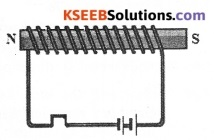Question 33.
What happens when a current-carrying conductor is placed in a magnetic field?
A current-carrying conductor experiences a mechanical force when placed in a magnetic field.

Question 34.
Mention the factors on which the mechanical force acting on a current-carrying conductor in a magnetic field depends.
The mechanical force on a current-carrying conductor in a magnetic field depends on:

1. The strength of the magnetic field,
2. The strength of the current flowing through the conductor, and
3. The angle between the direction of current and the direction of the magnetic field.

Question 35.
How do you show that a mechanical force acts on a conductor carrying current in a magnetic field?
Take a small aluminium rod AB about 5 cm long. Using two connecting wires suspend it horizontally from a stand, as shown in the figure. Place a strong horse-shoe magnet in such a way that the rod lies between the two poles with the magnetic field directed upwards. For this put the North Pole of the magnet vertically below and South Pole vertically above the aluminium rod.

Connect the aluminium rod in series with a battery, a key and a rheostat. Now pass current through the aluminium rod from end B to end A. It is observed that the rod is displaced towards the left. When the direction of current is reversed, the aluminium rod is now towards the right.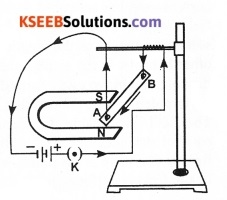This suggests that a mechanical force is exerted on the current-carrying aluminium rod when it is placed in a magnetic field. It also suggests that the direction of the mechanical force is also reversed when the direction of current through the conductor is reversed.

Question 36.
In the activity on the mechanical force on a conductor carrying current in a magnetic field, how do you think the displacement of rod AB will be affected if

1. Current in rod AB is increased;
2. A stronger horse-shoe magnet is used: and
3. Length of the rod AB is increased?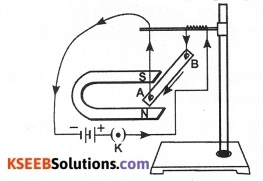1. When the current flowing through rod AB is increased, the rod AB gets displaced more due to an increase in the magnitude of the mechanical force acting on it.
2. When a stronger horse-shoe magnet is used in the activity, the mechanical force on the rod AB will increase and hence it gets displaced to a higher extent.
3. When the length of the rod is increased, the displacement of the rod will also get increased.Question 37.
What are the factors on which the direction of the mechanical force acting on a current carrying conductor placed in a magnetic field depends?
The direction of the mechanical force acting on a current-carrying conductor placed in a magnetic field depends on the

• Direction of flow of current, and the
• Direction of the magnetic field.

Question 38.
When will the magnitude of the mechanical force acting on a current-carrying conductor placed in a magnetic field become

1. Highest and
2. Lowest?

The magnitude of the mechanical force acting on a current-carrying conductor placed in a magnetic field:

1. Becomes highest when the direction of current is at right angles to the direction of the magnetic field.
2. Becomes lowest (zero) when the direction of current is parallel to the direction of the magnetic field.

Question 39.
When is the force experienced by a current-carrying conductor placed in a maenetic field largest?
The force experienced by a current-carrying conductor in a magnetic field is largest when the direction of current is perpendicular to the direction of the magnetic field.

Question 40.
Name the law that helps to find out the direction of the mechanical force on a current-carrying conductor in a magnetic field.
When the direction of current is at right angles to the direction of the magnetic field, the mechanical force acting on a current-carrying conductor in a magnetic field can be known by Fleming’s left hand rule.Question 41.
State Fleming’s left-hand rule.According to Fleming’s left-hand rule, ‘If we stretch the thumb, forefinger and middle finger of the left hand such that they are perpendicular to each other, if the forefinger points in the direction of magnetic field and the middle finger shows the direction of current, then, the thumb will point in the direction of motion of conductor.

Question 42.
Imagine that you are sitting in a chamber with your back to one wall. An electron beam, moving horizontally from the back wall towards the front wall, is deflected by a strong magnetic field to your right side. What is the direction of the magnetic field?
The direction of the magnetic field is vertically downward. The direction of current is from the front wall to the back wall because negatively charged electrons are moving from the back wall to the front wall. The direction of the magnetic force is rightward. Hence, using Fleming’s left hand rule, it can be concluded that the direction of the magnetic field inside the chamber is downward.

Question 43.
What is an electric motor? What is the principle of an electric motor?
A device that converts electrical energy into mechanical energy is called an electric motor. An electric motor works on the principle that a conductor carrying current experiences a mechanical force when placed in a magnetic field.

Question 44.
Draw a neat, labelled diagram of an electric motor.

OR

Draw the diagram of an electric motor and label the following parts:

1. Split rings,
2. Armature.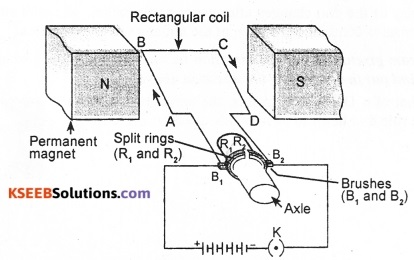B1 and B2 – Carbon brushes
Ba – BatteryQuestion 45.
What is a DC motor?
An electric motor that works on direct current (unidirectional current) is called a DC motor.

Question 46.
With the help of a neat diagram, explain the construction and working of a DC motor.

OR

Draw a labelled diagram of an electric motor. Explain its principle and working.
1. Construction:
A simple DC motor consists of a rectangular coil of wire ABCD mounted on an axle between the opposite poles N and S of a strong horseshoe magnet. One of the ends of the coil is soldered to a half of a split ring, say R1 while the other end is connected to the other half of the split ring R2 as shown in the figure. Two conducting carbon brushes B1 and B2 are kept pressed on R1 and R2 respectively. B] and B2 are connected to a source of direct current. The coil together with the split ring is called armature.

2. Working:
When a current is passed through the armature coil, a mechanical force acts on the limb AB and CD of the coil. These forces are equal in magnitude and opposite in direction. These two forces constitute a couple which rotates the coil between the magnetic poles.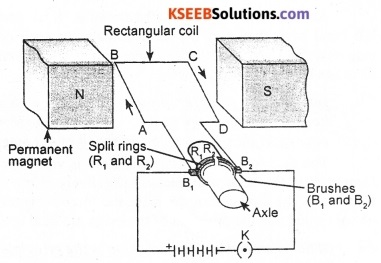As per the diagram, a current flows from the positive of the battery to the brush B1 and from B1 to R1. Therefore, current flows through the coil in the direction ABCD. The direction of the mechanical force acting on the limbs AB and CD can be known by Fleming’s left hand rule.

Obviously, the coil, during the first half of its cycle, rotates in anticlockwise direction. In the next half of its rotation, the brushes will be in contact with the other half of the split ring. This ensures the rotation of the coil in the same direction.

Question 47.
What is the function of a split ring in an electric motor?
In an electric motor, after every half rotation, the direction of current in the coil gets reversed due to change in orientation of the magnetic field. To ensure a continuous rotation, a split ring is attached to the coil so that the polarity of the coil changes after every half rotation. The split ring thus changes the direction of current and ensures continuous rotation of the armature in one direction.Question 48.
Identify the diagram given. What is the direction in which the armature rotates? Name the rule that helped you find out the answer to the question above.
The diagram is that of a DC motor. In this, the armature rotates in clockwise direction. The rule that helps to answer this question is Fleming’s left hand rule.Question 49.
How is the power of commercial electric motors enhanced?
The power of commercial electric motors is enhanced by:

1. Using an electromagnet in place of permanent magnet,
2. Taking large number of turns of the conducting wire in the current-carrying coil, and
3. Winding the coil on a soft iron core.

Question 50.
Name some devices in which electric motors are used.
Electric motor is used in all such devices where electric energy is required to be converted into ‘ mechanical energy. Such devices include electric fans, mixer grinders, coolers, air conditioners, washing machines, etc.

Question 51.
Who discovered that a changing magnetic field linked with a closed coil induces an electric current in the coil?
Michael Faraday discovered that a changing magnetic field linked with a closed coil induces an electric current in the coil.

Question 52.
Name the phenomenon discovered by Michael Faraday to produce electric current from a changing magnetic field.
Michael Faraday discovered a phenomenon called electromagnetic induction in which an electric current was produced in a coil using a changing magnetic field.

Question 53.
What is a galvanometer? How does it work?
A galvanometer is an instrument that can detect the presence of current in a circuit. In a galvanometer, gradations are made on either side of zero (the centre of the scale). The pointer remains at zero for zero current flowing through it.

It can deflect either to the left or to the right of the zero mark depending on the direction of current. Thus, a galvanometer not only detects the presence of current but also indicates the direction of flow of current.Question 54.
Describe Faraday’s magnet and coil experiment which led to the discovery of electromagnetic induction.

OR

Explain Faraday’s experiment to illustrate the phenomenon of electromagnetic induction.
Michael Faraday’s magnet and coil experiment consisted of an insulated copper coil having a large number of turns. He connected the ends of the coil to the terminals of a galvanometer. He thrust a pole of a strong bar magnet into the coil quickly.

He observed a momentary deflection in the galvanometer. He pulled the magnet out of the coil, The galvanometer now showed deflection in the opposite direction. He repeated this experiment with the other pole. The same effect was observed in the opposite direction.

However, there was no deflection in the galvanometer when the magnet was held at rest inside the coil. He kept the magnet at rest and moved the coil itself around the magnet. Even now, the galvanometer showed a deflection. Through this experiment, Faraday demonstrated that a changing magnetic field linked with a coil can produce electricity in the coil.Question 55.
Which was the generalization drawn from Faraday’s magnet and coil experiment on electromagnetic induction?
Faraday’s magnet and coil experiment showed that ‘whenever there is a relative motion between a closed coil and a magnetic field linked with it, an emf is induced inside the coil’.

Question 56.
You are given a coil of insulated copper wire and a bar magnet. How do you induce an electric current in the coil?
Connect the ends of the coil to a galvanometer. Now push a pole of the given magnet into the coil. The galvanometer shows a momentary deflection. On withdrawing the magnet from inside the coil, a deflection is seen in the galvanometer in the opposite direction. The deflection in the galvanometer shows that a current is induced in the coil.Question 57.
A bar magnet is placed inside a closed coil Both the magnet and the coil are made to move in the same direction with the same speed. Does this motion induce an emf in the coil? Why?
When a magnet and a coil move in the same direction with the same speed, the magnetic field linked with the coil does not change. Therefore, no emf is induced in the coil.

Question 58.
Can a stationary coil and stationary magnet produce induced current? Give reason.
A stationary coil and a stationary magnet cannot produce induced current. For current to get induced in a coil, the magnetic field linked with the coil should change. In the given situation, the magnetic field linked with the coil does not change. This is why no current is induced in the coil.

Question 59.
A coil of insulated copper wire is connected to a galvanometer. What would happen if a bar magnet is

1. Pushed into the coil,
2. Withdrawn from inside the coil,
3. Held stationary inside the coil?

1. Due to change in magnetic field in the coil, the galvanometer shows deflection.
2. Due to change in magnetic field in the coil, the galvanometer shows deflection in the opposite direction. It indicates the current in the coil is in opposite direction.
3. As the magnet is stationary, there is no change in magnetic field in the coil. Therefore, the galvanometer shows no deflection.

Question 60.
Explain different ways to induce current in a coil
Current can be induced in a coil by

1. Moving the coil in a magnetic field or moving the coil rapidly between the two poles of a horse-shoe magnet.
2. Bringing a bar magnet close to the coil or taking away from it.
3. Rotating the coil in a uniform magnetic field.

Question 61.
What is electromagnetic induction?
The process by which a changing magnetic field in a conductor induces a current in another conductor is called electromagnetic induction.

Question 62.
Name the device that works on the principle of electromagnetic induction.
An electric generator works on the principle of electromagnetic induction.Question 63.
What is meant by induced emf and induced current?
The emf produced in a coil during electromagnetic induction is called induced emf. The current that is induced in the coil is called induced current.

Question 64.
Mention the factors on which the emf induced in a coil by electromagnetic induction depends.
The current induced in a coil due to the changing magnetic field increases with the increase in:

1. The number of turns in the coil, and
2. The rate of change of magnetic field linked with the coil.

Question 65.
Electricity is induced in a coil by thrusting a bar magnet into the coil. What are the ways to increase the induced electric current in the coil?
The current induced in the coil can be increased by

1. Increasing the number of turns in the coil,
2. Taking a magnet of higher strength, and
3. Increasing the speed of motion of magnet inside the coil.

Question 66.
How do you demonstrate that any change in current in a coil will induce a current in another coil placed close to it?

OR

Describe Faraday’s coil and coil experiment.
Take two different coils (coil-1 and coil-2) of copper wire having turns 100 and 50 respectively. Insert them over a non-conducting cylinder as shown in the figure. Connect coil-1 in series with a battery, a rheostat and a plug key.

Also connect the other coil-2 to the terminals of a galvanometer. Close the key. Observe the galvanometer. The needle of the galvanometer instantly jumps to one side and just as quickly returns to zero, indicating a momentary current in coil-2.Disconnect coil-1 from the battery. The needle momentarily moves to the opposite side. It means that now the current flows in the opposite direction in coil-2. When the current in coil-1 is steady, no deflection is observed in the galvanometer. Whenever current through coil-1 is changed, a potential difference is induced in coil-2.Question 67.
In the figure, as the current changes in coil-1, the galvanometer connected to coil-2 shows deflection. Explain the phenomenon that causes this effect. Name and state the law used to know the direction of current in the device that works due to this phenomenon.
The process by which a changing magnetic field in a conductor conductor is called electromagnetic induction. Whenever the electric current through coil-1 changes, the magnetic field associated with it also changes. Thus the magnetic field lines associated with coil-2 also change. Hence the change in magnetic field lines associated with coil-2 is the cause of induced electric current in it.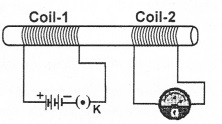The law used to know the direction of current in the device that works on this phenomenon is called Fleming’s right hand rule.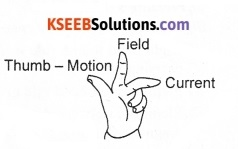Rule:
Fleming’s right-hand rule states that, if the fore finger, middle finger and the thumb of the right hand are stretched at right angles to each other, with the fore finger in the direction of magnetic field and the thumb in the direction of motion of the conductor, then the middle finger gives the direction of flow of induced current.

Question 68.
Why does current get induced in a coil when current flowing through another coil placed close to it is changed?
As the current in a coil changes, the magnetic field associated with it also changes. Thus the magnetic field lines around the second coil placed close to the first also change. Hence the change in magnetic field lines associated with the secondary coil is the cause of induced electric current in it.

Question 69.
Two circular coils A and B are placed close to each other. If the current in coil A is changed, will some current be induced in coil B? Give reason.
When the current in coil A is changed, some current will definitely be induced in coil B. When the current in coil A is changed, the magnetic field associated with it also changes. As a result, the magnetic field around coil B also changes. This change in magnetic field lines around coil B induces an electric current in it. This process is known as mutual (electromagnetic) induction.Question 70.
What rule helps us to find the direction of induced current?

OR

For what purpose is Fleming’s right hand rule used?
Direction of induced current in electromagnetic induction can be known by Fleming’s Right Hand Rule. This rule is also known as generator rule.Question 71.
When can we apply Fleming’s Right Hand Rule?
In electromagnetic induction, the induced current would be the highest when the direction of the magnetic field is perpendicular to the direction of motion of the coil. In this situation, Fleming’s Right Hand Rule can be applied to find out the direction of induced current.

Question 72.
State Fleming’s Right Hand Rule.
Extend the thumb, the fore finger and the middle finger of the right hand perpendicular to one another. If the fore finger shows the direction of magnetic field and the thumb shows the direction of motion of the coil, then, the middle finger will show the direction of induced current.Question 73.
How can you increase the induced current in a coil in the magnet and coil experiment on electromagnetic induction?
Induced current in a closed coil can be increased when

1. The magnet moves at a faster speed in and out of the coil
2. A stronger magnet is used
3. The number of turns in the coil is increased.

Question 74.
State the purpose for which the following rules are used:

1. Right hand thumb rule.
2. Fleming’s left hand rule.
3. Fleming’s right hand rule.

1. Right hand thumb rule is used to find the direction of the lines of magnetic force in a current-carrying wire.
2. Fleming’s left hand rule gives the direction of magnetic force acting on a conductor.
3. Fleming’s right hand rule shows the direction of induced current when a conductor moves in a magnetic field.Question 75.
State the rule to determine the direction of

1. A magnetic field produced around a straight conductor carrying current,
2. A force experienced by a current-carrying straight conductor placed in a masnetic field which is perpendicular to it, and
3. A current induced in a coil due to its rotation in a magnetic field.

1. Right hand thumb rule:
The rule states that, if you are holding a current-carrying straight conductor in your right hand such that the thumb points towards the direction of current, then, the fingers wrapped around the conductor will show the direction of the field lines of the magnetic field.

2. Fleming’s left hand rule:
‘If we stretch the thumb, fore finger and middle finger of the left hand at right angles to each other such that the fore finger points in the direction of magnetic field and the middle finger shows the direction of current, then, the thumb will point in the direction of the mechanical force acting on the conductor’.

3. Fleming’s right hand rule:
Extend the thumb, the fore finger and the middle finger of the right hand perpendicular to one another such that the fore finger shows the direction of magnetic field and the thumb shows the direction of motion of the coil. Then, the middle finger will show the direction of induced current.

Question 76.
What is alternating current? Represent alternating current graphically.
An electric current whose direction keeps on changing regularly is called alternating current. It is also called oscillating current. An alternating current is represented graphically as shown below: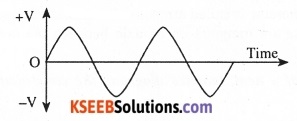Question 77.
Which sources produce alternatim current?
Dynamo or generators with slip rings will produce alternating current.Question 78.
What is direct current? Represent it graphically.
Electric current that flows through a conductor in only one direction is called direct current. The following is the graphical representation of direct current:Question 79.
Name some sources of direct current.
Battery, cells and generators that use split rings are some of the sources of direct current.

Question 80.
What is an electric generator? State the principle of an electric generator.
A device that converts mechanical energy into electrical energy is called an electric generator. A generator works on the principle of electromagnetic induction. It states that ‘whenever a magnetic field linked with a closed coil changes, an emf is induced inside the coil’.

Question 81.
Which are the two types of generators?
The two well-known types of generators are

1. AC generator and
2. DC generator.

Question 82.
Distinguish between alternating current (AC) and direct current (DC).
Current which changes direction after equal intervals of time is called alternating current. Current which does not change its direction with time is called direct current.

Question 83.
What is AC generator?
A generator that produces alternating current is called an AC generator.Question 84.
Draw a neat, labelled diagram of an AC generator.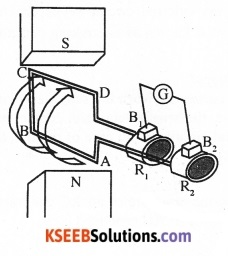• ABCD – Rectangular coil of wire
• N and S – Poles of a horse shoe magnet
• R1 and R2 – Thick metal rings
• B1 and B2 – Conducting brushes
• G – Galvanometer

Question 85.
List the major components of a simple AC generator.
A simple AC generator consists of a powerful horseshoe magnet, a rectangular coil of wire, two thick metal rings, two conducting brushes and a load.

Question 86.
What is the rotating part of a generator called? What does it consist of?
The rotating part of a generator is called armature. It consists of a rectangular coil of wire and two thick metal rings. Both of these are mounted on an axle between the opposite poles of the horseshoe magnet.

Question 87.
Explain with the help of a neat, labelled diagram, the construction and working of a simple AC generator.

OR

Explain the underlying principle and working of an electric generator by drawing a labelled diagram.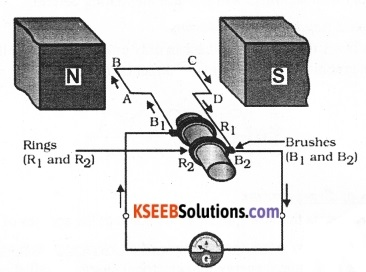1. Construction:
A simple AC generator consists of a rectangular coil of wire ABCD mounted on an axle between the opposite poles N and S of a strong horseshoe magnet. One of the ends of the coil is soldered to a thick metal ring, say R1 while the other end is connected to another similar ring R2 as shown in the figure.

These rings are called slip rings. Two conducting carbon brushes B1 and B2 are kept pressed on R1 and R2 respectively. B1 and B2 are connected to an external circuit. The coil together with the slip rings is called armature.2. Working:
A generator works on the principle of electromagnetic induction. The armature is rotated between the poles of the magnet. When the armature rotates, the magnetic field linked with the coil changes. This induces an electric current in the coil.

However, the current induced is different at different locations of the coil. During the first half rotation, a current is induced along DCBA if the rotation of the armature is anticlockwise. During this half cycle, current in the external circuit flows from B2 to B1.

During the second half rotation, current is induced along ABCD. Therefore, current in the external circuit flows from B1 to B2. Thus, the current in the external circuit keeps on changing its direction for every half rotation of the armature. Such a current is known as alternating current (AC) and the generator is known as AC generator.

Question 88.
What is the function of brushes in generators and motors?
The brushes in generators and motors conduct electric current between the stationary wires and the rotating parts of a motor or generator. In the case of generators, the brushes maintain contact between the slip rings (or split rings) and pass on the current to the external circuit. In the case of motors, the brushes pass the current from the source to the slip rings (or split rings).

Question 89.
What is meant by frequency of AC?
The number of complete rotations per second made by the armature of an AC generator is called the frequency of rotation of the armature. This is also the frequency of AC produced by it.

Question 90.
Mention the frequency of the electric current produced in India.
The frequency of electric current produced in India is 50 hertz (Hz).Question 91.
The armature of an AC generator rotates 100 times in 2 second. What is the frequency of rotation of the armature? What is the frequency of AC produced by this generator? How many times does the direction of AC change per second? Why?
The frequency of rotation is the number of complete rotations made by the armature in one second. Therefore, the frequency of rotation of the armature is 50 Hz. The frequency of rotation of the armature and the frequency of AC produced are same. Thus, the frequency of AC produced by the generator is also 50 Hz. The current changes its direction 100 times per second. This is because the direction of current changes twice for every rotation of the armature.

Question 92.
In a generator, when is the value of induced current lowest? When is the value of induced current highest? Give reason.
In a generator, the current induced is lowest when the coil is parallel to the direction of the magnetic field. The value of induced current is highest when the coil is perpendicular to the direction of the magnetic field.

When the plane of the coil is parallel to the direction of the magnetic field, the field lines do not intercept the coil. Therefore, the current induced is zero. When the plane of the coil is perpendicular to the direction of the magnetic field, the highest number of field lines intercept the coil. Therefore, the current induced is highest.

Question 93.
Draw a neat schematic diagram of a simple domestic electric circuit and name the parts.Question 94.
Describe briefly domestic electric circuit.
In our homes electricity is brought from the electric power station by two insulated cables made of aluminium or copper. Out of these two wires, one wire is in red insulation and is called ‘live wire’. The other wire is in black insulation and is called ‘neutral wire’.

The electricity coming into our house is actually alternating current of voltage 220 V. Both the live wire and the neutral wire enter in a box where a main fuse is put on the live wire. These two wires then pass through the electricity meter.

After the electric meter, these wires enter the distribution board through the main switch. The main switch is used to switch off the electricity supply whenever required. After the main switch, the live wire is connected to live wires of two separate circuits present in the house to supply electricity.

Out of these two circuits, one circuit of 5A rating, is used to run electric appliances with low power such as tube lights, bulbs and fans. The other circuit is of 15 A rating which is used to run electric appliances with higher power such as air conditioners, refrigerators, electric iron and heaters.It should be noted that all the circuits in the house are connected in parallel so that switching off of one circuit does not affect the other circuit. One more advantage of parallel connection of circuits is that each electric appliance gets equal voltage.

A third wire called ‘earth wire’, having green insulation, is usually connected to the body of metallic electric appliances. The earth wire sends the current from the body of the appliance to the earth whenever a live wire accidentally touches the body of metallic electric appliances.

Thus, the earth wire gives protection from severe electric shocks. Each electric appliance has a separate switch to switch on or switch off the flow of current to it.

Question 95.
Name two safety measures commonly used in electric circuits and appliances.
Two of the safety measures commonly used in electric circuits and appliances are:

• Earthing the metallic body of electrical appliances to protect from electric shocks.
• Use of electric fuse to protect circuit and appliances from the consequences of overloading.

Question 96.
What is the function of an earth wire? Why is it necessary to earth metallic appliances?
The earth wire is connected as a safety measure for all electrical appliances that have metallic body. It protects us from electric shocks due to accidental leakage of charges. The earth wire provides a low resistance-conducting path for electric current and passes the current to the earth.

Question 97.
All circuits are designed to carry a certain maximum value of current safely. The amount of current that can be safely drawn in a circuit depends on the power rating of the wire used there. When the amount of current passing through the circuit exceeds the maximum permissible limit, the wires get heated to such an extent that fire may be caused.Question 98.
When does an electric short-circuit occur? Explain.
An electric short-circuit occurs when the live wire and the neutral wire come in direct contact with each other. When this happens, the current flowing in the circuit rises alarmingly and short-circuiting occurs.

Short-circuiting happens when the insulation of wires is damaged and the live wire comes into contact with the neutral wire. When this happens, the resistance becomes too small leading to flow of large amount of current through the wires. Such a large current heats up the wires to such an extent that fire may be caused in the building or the gadgets used in the circuit may get damaged.

Question 99.
What precautions should be taken to avoid overloading of domestic electric circuits?

• Two separate circuits should be used in domestic wiring, one for lighting (5 A) and the other for heating (15 A).
• Fuse should be installed in both lighting and heating circuits.
• Parallel circuits should be used to operate gadgets.
• Current should not be drawn by plugging too many gadgets to a single plug point.

Question 100.
An electric oven of 2 kW power ratine is operated in a domestic electric circuit (220 V) that has a current ratine of 5 A, What result do you expect? Explain.
Given: Power P = 2 kW = 2000 W; Voltage = 220 V; Current rating of wires = 5 A.
Power, P = VI.
Current drawn by oven, I = $$\frac{\text { Power }}{\text { Voltage }}=\frac{2000 \mathrm{W}}{220 \mathrm{V}}$$ = 9.09 A.

Since the current drawn by the oven (9.09 A) is higher than the safe limit (5 A), there would be overloading. When the oven is operated, the fuse would blow off and the circuit would be broken. If no fuse is used in the circuit, there may be fire in the circuit.

Question 101.
How does overload and short-circuit occur in it an electric current? Explain. What is the function of fuse during this situation?
All electric circuits are designed to carry a certain maximum value of current safely. Over-loading occurs when the current value exceeds the safe limit of the circuit. Over-loading can occur due to short- circuiting or faulty electric appliance.

Short-circuit occurs when the live wire and the neutral wire come into direct contact instead of passing through a load leading to a huge upsurge of current. This is also an instance of overloading of the circuit. A fuse protects the circuit and its appliances in the event of overloading as well as short circuit.Question 102.
State whether the following statements are true or false:

1. An electric motor converts mechanical energy into electrical energy..
2. An electric generator works on the principle of electromagnetic induction.
3. The field at the centre of a lone circular coil carrying current will be parallel straight lines.
4. A wire with a green insulation is usually the live wire of an electric supply.

1. False
2. True
3. True
4. False.

Fill In The Blanks

1. The phenomenon of electromagnetic induction was discovered by Faraday
2. A coil of many turns wound in the form of a cylinder is called solenoid
3. The direction of magnetic field around a current-carrying conductor can be known by Right hand thumb rule
4. The maximum load of current in heating circuits in our homes is 15 A
5. A device that converts electrical energy into mechanical energy is called electric motor
6. A generator works on the principle of electromagnetic induction
7. The insulation colour of the earth wire in domestic circuits is green
8. A device that detects electric current in a circuit is galvanometerMultiple Choice Questions

Question 1.
The magnetic field inside a long straight solenoid carrying current
(A) is zero.
(B) decreases as we move towards its end.
(C) increases as we move towards its end.
(D) is same at all points.
(D) is same at all points.

Question 2.
An electron enters a magnetic field at right angles to it as shown in the figure. The direction of force acting on the electron will be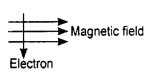(A) to the right.
(B) to the left.
(C) out of the page.
(D) into the page.
(D) into the page.Question 3.
Which of the following properties of a proton can change while it moves freely in a magnetic field? (There may be more than one correct answer.)
(A) mass
(B) speed
(C) velocity
(D) momentum
(D) momentum

Question 4.
A positively-charged particle (alpha particle) projected towards west is deflected towards north by a magnetic field. The direction of magnetic field is
(A) towards south
(B) towards east
(C) downward
(D) upward
(C) downward

Question 5.
A rectangular coil of copper wires is rotated in a magnetic field. The direction of the induced current changes once in each
(A) one-fourth revolution
(B) half revolution
(C) one revolution
(D) two revolutions
(B) half revolution

Question 6.
Which of the following correctly describes the magnetic field near a long straight wire?
(A) The field consists of straight lines perpendicular to the wire.
(B) The field consists of straight lines parallel to the wire.
(C) The field consists of radial lines originating from the wire.
(D) The field consists of concentric circles centred on the wire.
(D) The field consists of concentric circles centred on the wire.Question 7.
The phenomenon of electromagnetic induction is
(A) the process of charging a body.
(B) the process of generating magnetic field due to a current passing through a coil.
(C) the process of producing induced current in a coil due to relative motion between magnet and the coil.
(D) the process of rotating the coil of an electric motor.
(C) the process of producing induced current in a coil due to relative motion between magnet and the coil.

Question 8.
The most important safety method used for protecting home appliances from short-circuiting or overloading is
(A) earthing
(B) use of fuse
(C) use of stabilizers
(D) use of electric meter
(B) use of fuse

Question 9.
The device used for producing electric current is called a
(A) generator.
(B) galvanometer.
(C) ammeter.
(D) motor.
(A) generator.

Question 10.
The essential difference between an AC generator and a DC generator is that
(A) AC generator has an electromagnet while a DC generator has permanent magnet.
(B) DC generator will generate a higher voltage.
(C) AC generator will generate a higher voltage.
(D) AC generator has slip rings while the DC generator has a commutator.
(D) AC generator has slip rings while the DC generator has a commutator.Question 11.
At the time of short-circuit, the current in the circuit
(A) reduces substantially.
(B) does not change.
(C) increases heavily.
(D) varies continuously.
(C) increases heavily.

Question 12.
Commercial electric motors do not use
(A) a soft iron core on which the coil is wound.
(B) a permanent magnet to rotate the armature.
(C) an electromagnet to rotate the armature.
(D) effectively large number of turns of conducting wire in the current-carrying coil.
(B) a permanent magnet to rotate the armature.

Question 13.
Choose the incorrect statement from among the following:
(A) Fleming’s right-hand rule is a simple rule to know the direction of induced current.
(B) The right-hand thumb rule is used to find the direction of magnetic field due to current carrying conductor.
(C) The difference between direct and alternating currents is that direct current always flows in one direction, whereas alternating current reverses its direction periodically.
(D) In India, the AC changes direction after every 1/50 second.
(D) In India, the AC changes direction after every 1/50 second.

Question 14.
Choose the incorrect statement from the following regarding magnetic field lines:
(A) If magnetic field lines are parallel and equidistant, they represent zero field strength.
(B) Magnetic field lines are closed curves.
(C) Relative strength of magnetic field is shown by the degree of closeness of the field lines.
(D) The direction of magnetic field at a point is taken to be the direction in which the north pole of a magnetic compass needle points.
(A) If magnetic field lines are parallel and equidistant, they represent zero field strength.Question 15.
Which of the following has magnetic field like that of the one around a bar magnet?
(A) Current-carrying wire
(B) Current-carrying ring
(C) Current-carrying solenoid
(D) Current-carrying rectangular loop
(C) Current-carrying solenoid

Question 16.
Who gave the principle of electromagnetic induction?
(B) Oersted
(C) Volta
(D) Ampere

Question 17.
Which is the direction of mechanical force acting on a current-carrying wire placed in a magnetic field?
(A) Along magnetic field and electric field.
(B) Along the electric current but opposite the magnetic field.
(C) Perpendicular to magnetic field and the direction of current.
(D) Opposite to magnetic field.
(C) Perpendicular to magnetic field and the direction of current.

Question 18.
To convert an AC generator into a DC generator
(A) split-ring type commutator must be used
(B) slip rings and brushes must be used
(C) a stronger magnetic field has to be used
(D) a rectangular wire loop has to be used
(A) split-ring type commutator must be used

Question 19.
The upper limit of current that can safely flow through the lighting and heating circuit in our houses is respectively
(A) 5 A, 10 A
(B) 5 A, 15 A
(C) 10 A, 15 A
(D) 15 A, 5 A
(B) 5 A, 15 AQuestion 20.
A commutator changes the direction of current in the coil of
(A) a DC motor only
(B) a DC motor and an AC generator
(C) a DC motor and a DC generator
(D) an AC generator only.